# TStools for R

By | April 19, 2014

This is a collection of functions for time series analysis/modelling for R. Follow link to GitHub. If you need help installing this package in R have a look at this post. Alternatively just type in R the following commands:

```> if (!require("devtools"))
install.packages("devtools")
> devtools::install_github("trnnick/TStools")
```

At the time of posting the following functions are included:

• cmav: Centred moving average;
• coxstuart: Cox-Stuart test;
• decomp: Time series decomposition;
• kdemode: Mode estimation via KDE;
• nemenyi: Friedman and Nemenyi tests;
• regopt: Identify regression beta using various cost functions;
• seasplot: Seasonal plots.

Here are some examples of what these functions do.

The cmav function will calculate the centred moving average of a time series. It differs from the ma function in the forecast package in the sense that it can provide values for the first and last segment of the time series, where the centred moving average cannot be calculated, by fitting an ETS model.

`> cmav(referrals,outplot=TRUE)`The decomp function performs classical decomposition on a time series.

```> decomp(referrals,outplot=TRUE)
```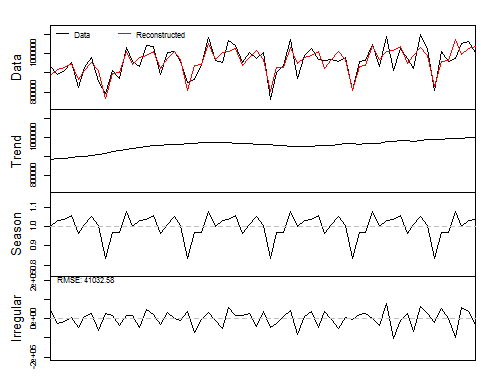You can estimate the season using either the mean or the median of the historical indices or fit a pure seasonal model. Here, I also choose the type of decomposition and ask the seasonal indices for the next 12 months.

```> decomp(referrals,outplot=TRUE,type="pure.seasonal",decomposition="additive",h=12)
```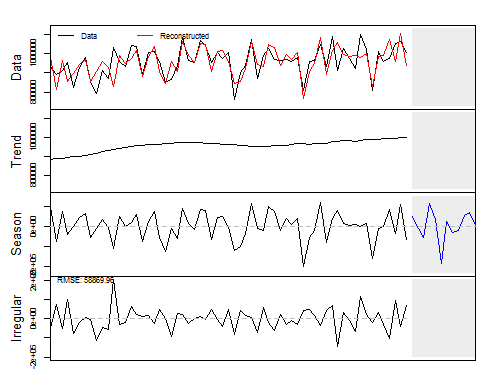The kdemode can be used to find the mode of a distribution by kernel density estimation. The bandwidth is automatically identified.

```> data <- rlnorm(200,mean=0,sd=1)
kdemode(data,outplot=TRUE)```The bandwidth is estimated automatically using the excellent estimation proposed by Botev et al. Alternatively the bandwidth can be calculated using either the Sheater and Jones method or the Silverman heuristic. This was quite useful for producing the mode ensemble neural networks that are proposed here.

The nemenyi function can be used to run the Nemenyi test to compare models. Here is an example:

```> N <- 50
> M <- 4
> data <- matrix( rnorm(N*M,mean=0,sd=1), N, M)
> data[,2] <- data[,2]+1
> data[,3] <- data[,3]+0.7
> data[,4] <- data[,4]+0.5
> colnames(data) <- c("Method A","Method B","Method C","Method D");
> nemenyi(data,conf.int=0.95,plottype="vline")
```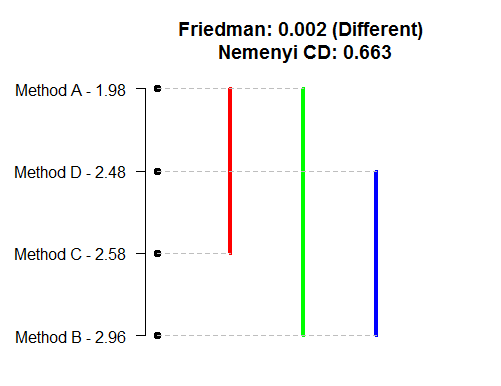I can also get the MCB test by using:

```> nemenyi(data,conf.int=0.95,plottype="mcb")
```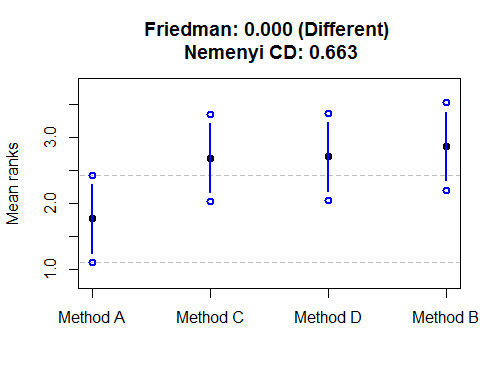These tests use the same statistic. A discussion on this and various visualisations can be found here.

Finally, the seasplot function produces seasonal plots. It differs from the similar seasonplot in the forecast package as it can automatically test for presence of trend and remove it, if present.

```> seasplot(referrals)
```Furthermore, there are a few alternative visualisations you can do with seasplot. Here are some examples: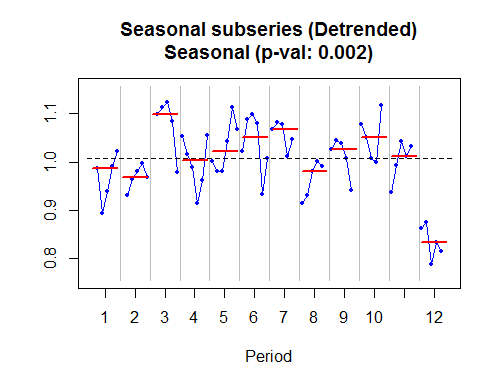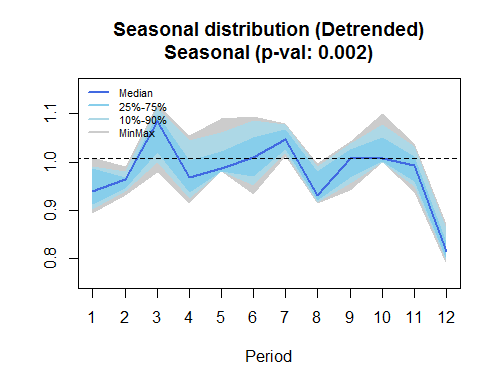You may wonder how is that p-value calculated. Based on the definition of deterministic seasonality, I just compare the distributions of the seasonal elements and test whether at least one is different. I do this by using the nonparametric Friedman test.

The plan is to keep on updating TStools on GitHub. When there are substantial updates, I will post them here.

For examples of some of the new features of TStools have a look here.

## 7 thoughts on “TStools for R”

1.Dmitry

Hi Nikos,
I am interested in your seasplot() function. In particular, seasonal distribution option.
I wonder, is it possible to extract somehow those median values of seasonal indices that are shown in the plot?
Thank you!

1.Nikos Post author

You will need some additional calculations, here is an example. Let us first get the seasonal plot for a time series (I will use AirPassengers).
`seas < - seasplot(AirPassengers)`
The output seas contains the seasonal indices, from which we can calcuate means, medians, percentiles or whatever we need. For example for medians:
`apply(season\$season,2,median,na.rm=TRUE)`
or for the 80% percentile:
`apply(season\$season,2,quantile,prob=0.8,na.rm=TRUE)`
You can change the prob argument to get any percentile that you need.
Hope this helps!
Nikos

1.Dmitry

Thank you Nikos, it helped!
Just a minor comment, in your code above should it be
apply(seas\$season,2,median,na.rm=TRUE) instead of apply(season\$season,2,median,na.rm=TRUE)?

2.RS

Hi,

I am getting the following error while trying to install TSTools:

ERROR: dependency ‘smooth’ is not available for package ‘TStools’

Thanks!

1.Nikos Post author

I need to check it, meanwhile try installing the smooth package from CRAN first, and then TStools. That should get it resolved.

3.jingjing

Hi,
Could you please tell me where can I find the explanation of the picture of nemenyi test

1.Nikos Post author

The visualisation is based on this paper: Demšar, Janez. “Statistical comparisons of classifiers over multiple data sets.” Journal of Machine learning research 7.Jan (2006): 1-30.You may find this presentation useful that uses a similar visualisation: http://kourentzes.com/forecasting/2012/04/19/statistical-significance-of-forecasting-methods-an-empirical-evaluation-of-the-robustness-and-interpretability-of-the-mcb-anom-and-friedman-nemenyi-test/